How capacitors in Parallel & Series ere like.
2022-12-26

Capacitors in parallel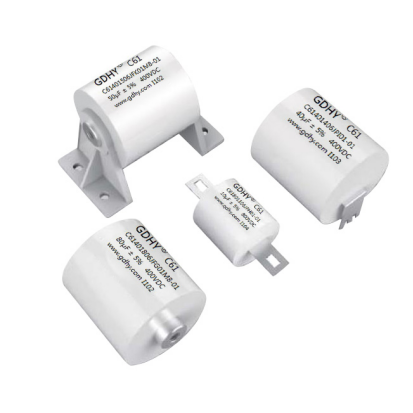Capacitors, like other electrical elements, can be connected to other elements either in series or in parallel. Sometimes it is useful to connect several capacitors in parallel in order to make a functional block such as the one in the figure. In such cases, it is important to know the equivalent capacitance of the parallel connection block. This article will focus on analyzing the parallel connection of capacitors and possible applications for such circuits.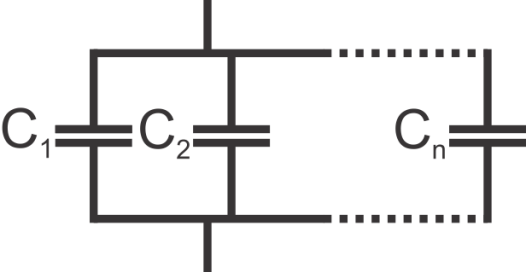Analysis

All capacitors in the parallel connection have the same voltage across them, meaning that:

equal voltages

where V1 to Vn represent the voltage across each respective capacitor. This voltage is equal to the voltage applied to the parallel connection of capacitors through the input wires. However, the amount of charge stored at each capacitor is not the same, and depends on the capacitance of each capacitor according to the formula:

where Qn is the amount of charge stored on a capacitor, Cn is the capacitance of the capacitor and Vn is the voltage applied to the capacitor, which is equal to the voltage applied to the complete parallel connection block. The total amount of charge that is stored by the block of capacitors is represented by Q and is divided between all the capacitors present in this circuit. This is represented by:

The following equation is used to determine the equivalent capacitance for the parallel connection of multiple capacitors:

where Ceq is the equivalent capacitance of the parallel connection of capacitors, V is the voltage applied to the capacitors through the input wires, and Q1 to Qn represent the charges stored at each respective capacitor. This brings us to the important conclusion that:

which means that the equivalent capacitance of the parallel connection of capacitors is equal to the sum of the individual capacitances. This result is intuitive as well - the capacitors in parallel can be regarded as a single capacitor whose plate area is equal to the sum of plate areas of individual capacitors.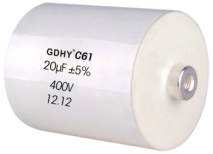Applications

Capacitors are devices used to store electrical energy in the form of electrical charge. By connecting several capacitors in parallel, the resulting circuit is able to store more energy since the equivalent capacitance is the sum of individual capacitances of all capacitors involved. This effect is used in some applications.

DC power supplies

One example are DC supplies which sometimes use several parallel capacitors in order to better filter the output signal and eliminate the AC ripple. By using this approach, it is possible to use smaller capacitors that have superior ripple characteristics while obtaining higher capacitance values.

Higher capacitance values

There are some applications which simply require capacitance values which are much higher than commercially available capacitors are able to offer. Capacitor banks are used in such applications. One example are capacitor banks used for power factor correction with inductive loads. Another example are energy storage capacitor banks which are announced for use in automotive industry, namely KERS (Kinetic Energy Recovery System) used for regenerative braking in large vehicles such as trams, as well as hybrid cars.

One high magnetic field laboratory powers the worlds most powerful magnet capable of delivering a magnetic field of almost 100 teslas by storing energy in a capacitor bank. The stored energy is released through a magnetic coil in a very short amount of time, generating a very powerful magnetic field.

In any case, capacitor banks can reach very high capacitance values. By using several supercapacitors connected in parallel, capacitances of several tens of kilofarads are feasible, especially bearing in mind that supercapacitors are capable of achieving capacitance values of over 2000 farads.

Design restrictions

When connecting capacitors in parallel, there are some points to keep in mind. One is that the maximum rated voltage of a parallel connection of capacitors is only as high as the lowest voltage rating of all the capacitors used in the system. Thus, if several capacitors rated at 500V are connected in parallel to a capacitor rated at 100V, the maximum voltage rating of the complete system is only 100V, since the same voltage is applied to all capacitors in the parallel circuit.

Safety

Another point to keep in mind is that capacitor banks can be dangerous due to the amount of energy stored and the fact that capacitors are able to release the stored energy in a very short amount of time. This stored energy is sometimes efficient to cause serious injuries or damage to electrical wiring and devices if shorted out by accident.

Capacitors in series

Capacitors in series

Like other electrical elements, capacitors serve no purpose when used alone in a circuit. They are connected to other elements in a circuit in one of two ways: either in series or in parallel. In some cases it is useful to connect several capacitors in series in order to make a functional block: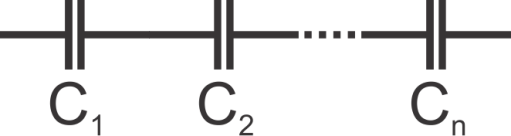Analysis

When this block is connected to a voltage source, each capacitor in the block stores an equal amount of charge, which means that the total amount of charge is evenly distributed across all of the capacitors, regardless of their capacitance. The amount of charge stored at each capacitor equals:

equal charges

where Qtotal is the total amount of charge in the complete block, and Q1 to Qn are charges at each individual capacitor.

In order to explain why the charges at every capacitor are mutually equal, and equal to the total amount of charge stored in the complete series connection block, let us assume that all capacitors were uncharged at one point in time. When voltage is first applied across the block, the same current flows through all the capacitors and as a result, charge shift occurs. Electrons are carried from one plate of each capacitor to the other, which means that the charge stored by a plate of any of the capacitors must have come from the adjacent capacitors plate. This means that charge carriers (electrons) have simply shifted through all the capacitors, which is the reason that the charges at each capacitor are equal.

That being said, it must be noted that the voltages across each capacitor are not equal, and are calculated for each capacitor by using the known formula:

voltage

where Qn is the amount of charge on every capacitor in the series connection, Cn is the capacitance of the capacitor, and Vn is the voltage across the capacitor.

By applying the Kirchoffs Voltage Law to the series connection block, the voltage across the block equals the sum of the voltages across individual capacitors:

KVL

By substituting the above formula for the voltage across a capacitor into this equation, having in mind that the charge at every capacitor equals the total charge stored in the series connection, we obtain the following formula:

equivalent1

which gives:

equivalent

where Ceq is the equivalent capacitance, and C1 to Cn are the capacitance values of every individual capacitor in the series connection.

In other words, the reciprocal value of the equivalent capacitance is equal to the sum of reciprocal capacitance values for each capacitor in the series connection.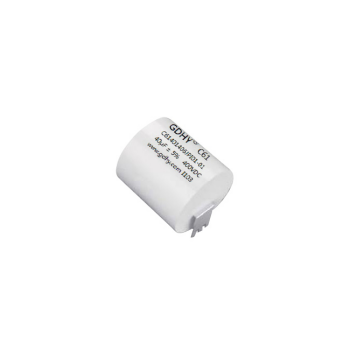Applications

Capacitive voltage divider

A voltage divider is a device which divides the applied voltage into two or more voltage outputs at a given ratio. They can be constructed using resistors or reactive elements such as capacitors. When capacitors are connected in series and a voltage is applied across this connection, the voltages across each capacitor are generally not equal, but depend on the capacitance values.

More precisely, the ratio of the voltages across individual capacitors is the inverse of the ratio of the capacitance values of each individual capacitor in the series. It should be noted that for practical purposes, capacitive voltage dividers are only useful in AC circuits, since capacitors do not pass DC signals, and also because they have a certain voltage leakage. The following figure illustrates the capacitive voltage divider: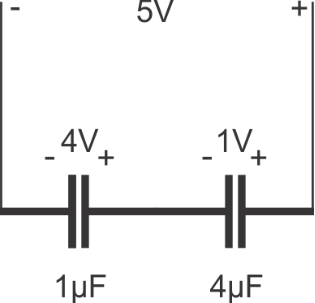Higher working voltage

It is sometimes desirable to use a series connection of capacitors in order to be able to work with higher voltages. For example, let us assume that a 5kV power supply needs to be filtered using capacitors, and that the only available capacitors are rated at 1kV and are all of identical capacitance values. In this case, by connecting five or more such capacitors in series, the high voltage would be divided across all the capacitors and the maximum rating would not be exceeded.

Another example for the use of serially connected capacitors is a possible replacement of a car battery with a capacitor bank made of supercapacitors. Since their maximum rated voltage is only about 2.7 volts, by connecting six such supercapacitors in series, the operating voltage of the bank is raised to levels acceptable for automotive use. The advantage to using supercapacitors over a battery is the weight benefit, however this technology still needs improvement due to leakage currents and much higher capacities offered by batteries.

Safety

A certain safety margin should be introduced when using the series capacitor circuit, and more than five capacitors would be used in a good design for this example due to two reasons. The first reason is that capacitors have a certain capacitance tolerance, and they cannot be made to identical capacitance values. As a consequence, a capacitor with a slightly smaller capacitance value would be exposed to a slightly larger voltage than the maximum rate voltage in this example. The second reason is that in reality capacitors sometimes fail. If one of the capacitors failed and became a short-circuit, the applied voltage of 5kV would be divided across the remaining 4 capacitors rated at only 1kV, and this would cause a cascade of failures, eventually shorting the voltage source and resulting in the complete destruction of the filter.

RECOMMENDED FOR YOU
GDHY Revolutionizing Capacitor Design to Enhance Performance and Efficiency in Small Welding and Quenching Equipment
Precautions for using film capacitors
Start capacitor in AC Induction Motor
How does metallized polyester film capacitors work in the application?
How to chose water cooled resonant capacitor ?
What is an axial film capacitor?
What is a plastic film capacitor?
IGBT Snubber Capacitors Selection Guide
What is a metallized polyester film capacitor?
What is a coupling capacitor and a bypass capacitor?
Get In Touch With Us
If you contact us now for more details, you can take samples for free. Our service team will get back to you with in 24 hours normally !